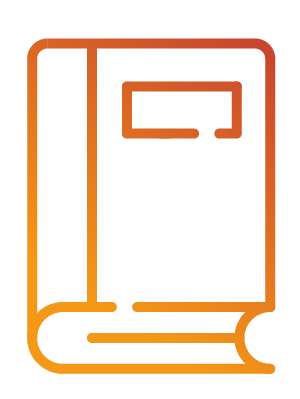Système d’information en philosophie des sciencesInformation system on philosophy of science

ImprimerEnvoyer le lien

MONOGRAPHIEAn Introduction to Non-Classical Logic. From If to Is

Résumé :

Anglais

This revised and considerably expanded 2nd edition, published in 2008, brings together a wide range of topics, including modal, tense, conditional, intuitionist, many-valued, paraconsistent, relevant, and fuzzy logics. Part 1, on propositional logic, is the old Introduction, but contains much new material. Part 2 is entirely new, and covers quantification and identity for all the logics in Part 1. The material is unified by the underlying theme of world semantics. All of the topics are explained clearly using devices such as tableau proofs, and their relation to current philosophical issues and debates are discussed. Students with a basic understanding of classical logic will find this book an invaluable introduction to an area that has become of central importance in both logic and philosophy. It will also interest people working in mathematics and computer science who wish to know about the area. – Contents : Preface to the first edition; Preface to the second edition; Mathematical prolegomenon. – Part I. Propositional Logic: – 1. Classical logic and the material conditional; – 2. Basic modal logic; – 3. Normal modal logics; – 4. Non-normal modal logics; strict conditionals; – 5. Conditional logics; – 6. Intuitionist logic; – 7. Many-valued logics; – 8. First degree entailment; – 9. Logics with gaps, gluts, and worlds; – 10. Relevant logics; – 11. Fuzzy logics; 11a. Appendix: Many valued modal logics; Postscript: An historical perspective on conditionals. – Part II. Qualification and Identity: – 12. Classical logic; – 13. Free logic; – 14. Constant domain modal logics; – 15. Variable domain modal logics; – 16. Necessary identity in modal logic; – 17. Contingent identity in modal logic; – 18. Non-normal modal logics; – 19. Conditional logics; – 20. Intuitionist logic; – 21. Many-valued logics; – 22. First degree entailment; – 23. Logics with gaps, gluts, and worlds; – 24. Relevant logics; – 25. Fuzzy logics. – Postscript: A methodological coda. – Includes bibliographical references (p. 587-602) and index.